Home
Hostname: page-component-78bd46657c-9sqjz Total loading time: 0.433 Render date: 2021-05-06T08:00:15.674Z Has data issue: true Feature Flags: { "shouldUseShareProductTool": true, "shouldUseHypothesis": true, "isUnsiloEnabled": true, "metricsAbstractViews": false, "figures": false, "newCiteModal": false, "newCitedByModal": true }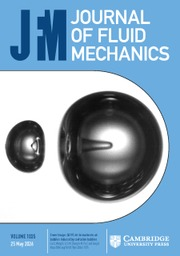Journal of Fluid Mechanics

# On a suspension of nearly spherical colloidal particles under large-amplitude oscillatory shear flow

Published online by Cambridge University Press:  22 February 2016

## Abstract

The dynamics of a dilute, monodisperse suspension of nearly spherical particles that undergo Brownian rotations in an oscillatory simple shear flow is quantified, as a paradigm for large-amplitude oscillatory shear (LAOS) rheology of complex fluids. We focus on the ‘strongly nonlinear’ regime of LAOS, defined by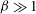${\it\beta}\gg 1$ and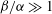${\it\beta}/{\it\alpha}\gg 1$ , where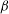${\it\beta}$ is a dimensionless shear rate (or Weissenberg number) and${\it\alpha}$ is a dimensionless oscillation frequency (or Deborah number). We derive an asymptotic solution for the long-time periodic orientation probability density function of the particles. Our analysis reveals that the orientation dynamics consists of ‘core’ regions of rapid oscillation (on the time scale of the inverse of the shear-rate amplitude), separated by comparatively short ‘turning’ regions of slow evolution when the imposed flow vanishes. Uniformly valid approximations to the shear stress and normal stress differences (NSDs) of the suspension are then constructed: the non-Newtonian contribution to the shear stress, first NSD and second NSD, decays as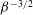${\it\beta}^{-3/2}$ ,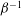${\it\beta}^{-1}$ and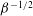${\it\beta}^{-1/2}$ , respectively, at large${\it\beta}$ . These stress scalings originate from the orientation dynamics at the turning regions. Therefore, it is the occasions when the flow vanishes that dominate the rheology of this paradigmatic complex fluid under LAOS.

## JFM classification

Type
Rapids
Information
Journal of Fluid Mechanics , 25 March 2016 , R5

## Access options

Get access to the full version of this content by using one of the access options below.

## References

Aouane, O., Thiébaud, M., Benyoussef, A. & Wagner, C. 2014 Vesicle dynamics in a confined Poiseuille flow: from steady state to chaos. Phys. Rev. E 90, 033011.CrossRefGoogle Scholar
Adrian, D. W. & Giacomin, A. J. 1992 The quasiperiodic nature of a polyurethane melt in oscillatory shear. J. Rheol. 36, 12271243.CrossRefGoogle Scholar
Bharadwaj, N. A. & Ewoldt, R. H. 2014 The general low-frequency prediction for asymptotically nonlinear material functions in oscillatory shear. J. Rheol. 58, 891910.CrossRefGoogle Scholar
Bird, R. B., Warner, H. R. Jr & Evans, D. C. 1971 Kinetic theory and rheology of dumbbell suspensions with Brownian motion. Adv. Polym. Sci. 8, 190.CrossRefGoogle Scholar
Brenner, H. & Condiff, D. W. 1974 Transport mechanics in systems of orientable particles. IV. Convective transport. J. Colloid Interface Sci. 47, 199264.CrossRefGoogle Scholar
Ewoldt, R. H., Hosoi, A. E. & McKinley, G. H. 2008 New measures for characterizing nonlinear viscoelasticity in large amplitude oscillatory shear. J. Rheol. 52, 14271458.CrossRefGoogle Scholar
Frattini, P. L. & Fuller, G. G. 1986 Rheo-optical studies of the effect of weak Brownian rotations in sheared suspension. J. Fluid Mech. 168, 119150.CrossRefGoogle Scholar
Giacomin, A. J., Bird, R. B., Johnson, L. M. & Mix, A. W. 2011 Large-amplitude oscillatory shear flow from the corotational Maxwell model. J. Non-Newtonian Fluid Mech. 166, 10811099.CrossRefGoogle Scholar
Goddard, J. D. & Miller, C. 1967 Nonlinear effects in the rheology of dilute suspensions. J. Fluid Mech. 28, 657673.CrossRefGoogle Scholar
Graham, M. D. 1995 Wall slip and the nonlinear dynamics of large amplitude oscillatory shear flows. J. Rheol. 39, 697712.CrossRefGoogle Scholar
Gurnon, A. K. & Wagner, N. J. 2012 Large amplitude oscillatory shear (LAOS) measurements to obtain constitutive equation model parameters: Giesekus model of banding and nonbanding wormlike micelles. J. Rheol. 56, 333351.CrossRefGoogle Scholar
Hatzikiriakos, S. G. & Dealy, J. M. 1991 Wall slip of molten high density polyethylene. I. Sliding plate rheometer studies. J. Rheol 35, 497523.CrossRefGoogle Scholar
Hinch, E. J. 1991 Perturbation Methods. Cambridge University Press.CrossRefGoogle Scholar
Hyun, K., Kim, S. H, Ahn, K. H. & Lee, S. J. 2002 Large amplitude oscillatory shear as a way to classify the complex fluids. J. Non-Newtonian Fluid Mech. 107, 5165.CrossRefGoogle Scholar
Hyun, K. & Wilhelm, M. 2009 Establishing a new mechanical nonlinear coefficient Q from FT-rheology: first investigation of entangled linear and comb polymer systems. Macromolecules 42, 411422.CrossRefGoogle Scholar
Hyun, K., Wilhelm, M., Klein, C. O., Cho, S. K., Nam, J. G., Ahn, K. H., Lee, S. J., Ewoldt, R. H. & McKinley, G. H. 2011 A review of nonlinear oscillatory shear tests: analysis and application of large amplitude oscillatory shear (LAOS). Proc. Polym. Sci. 36, 16971753.CrossRefGoogle Scholar
Jeffery, G. B. 1922 The motion of ellipsoidal particles immersed in a viscous fluid. Proc. R. Soc. Lond. A 102, 161179.CrossRefGoogle Scholar
Khair, A. S. 2016 Large amplitude oscillatory shear of the Giesekus model. J. Rheol. 60, 257266.CrossRefGoogle Scholar
Larson, R. G. 1999 The Structure and Rheology of Complex Fluids. Oxford University Press.Google Scholar
Leahy, B. D., Koch, D. L. & Cohen, I. 2015 The effect of shear flow on the rotational diffusion of a single axisymmetric particle. J. Fluid Mech. 772, 4279.CrossRefGoogle Scholar
Leal, L. G. & Hinch, E. J. 1972 The rheology of a suspension of nearly spherical particles subject to Brownian rotations. J. Fluid Mech. 55, 745765.CrossRefGoogle Scholar
Onogi, S., Masuda, T. & Matsumoto, T. 1970 Non-linear behavior of viscoelastic materials. I. Disperse systems of polystyrene solution and carbon black. Trans. Soc. Rheol. 14, 275294.CrossRefGoogle Scholar
Pearson, D. S. & Rochefort, W. E. 1982 Behavior of concentrated polystyrene solutions in large-amplitude oscillating shear fields. J. Polym. Sci. B 20, 8398.Google Scholar
Philippoff, W. 1966 Vibrational measurements with large amplitudes. Trans. Soc. Rheol. 10, 317334.CrossRefGoogle Scholar
Rallison, J. M. 1980 Note on the time-dependent deformation of a viscous drop which is almost spherical. J. Fluid Mech. 98, 625633.CrossRefGoogle Scholar
Rogers, S. A., Erwin, B. M., Vlassopoulos, D. & Cloitre, M. 2011 A sequence of physical processes determined and quantified in LAOS: application to a yield stress fluid. J. Rheol. 55, 435458.CrossRefGoogle Scholar
Rogers, S. A. & Lettinga, M. P. 2012 A sequence of physical processes determined and quantified in large-amplitude oscillatory shear (LAOS): application to theoretical nonlinear models. J. Rheol. 56, 125.CrossRefGoogle Scholar
Russel, W. B. 1978 Bulk stresses due to deformation of the electrical double layer around a charged sphere. J. Fluid Mech. 85, 673683.CrossRefGoogle Scholar
Swan, J. W., Furst, E. M. & Wagner, N. J. 2014 The medium amplitude oscillatory shear of semi-dilute colloidal suspensions. Part I: Linear response and normal stress differences. J. Rheol. 58, 307337.CrossRefGoogle Scholar
Vermant, J., Yang, H. & Fuller, G. G. 2001 Rheooptical determination of aspect ratio and polydispersity of nonspherical particles. AIChE J. 47, 790798.CrossRefGoogle Scholar
Vlahovska, P. M., Blawzdziewicz, J. & Loewenberg, M. 2002 Nonlinear rheology of a dilute emulsion of surfactant-covered spherical drops in time-dependent flows. J. Fluid Mech. 463, 124.CrossRefGoogle Scholar
Wilhelm, M. Fourier-transform rheology. Macromol. Mater. Engng 287, 83105.3.0.CO;2-B>CrossRefGoogle Scholar
Young, Y.-N., Blawzdziewicz, J., Cristini, V. & Goodman, R. H. 2008 Hysteretic and chaotic dynamics of viscous drops in creeping flows with rotation. J. Fluid Mech. 607, 209234.CrossRefGoogle Scholar

# Send article to Kindle

Note you can select to send to either the @free.kindle.com or @kindle.com variations. ‘@free.kindle.com’ emails are free but can only be sent to your device when it is connected to wi-fi. ‘@kindle.com’ emails can be delivered even when you are not connected to wi-fi, but note that service fees apply.

Find out more about the Kindle Personal Document Service.

On a suspension of nearly spherical colloidal particles under large-amplitude oscillatory shear flow
Available formats
×

# Send article to Dropbox

To send this article to your Dropbox account, please select one or more formats and confirm that you agree to abide by our usage policies. If this is the first time you use this feature, you will be asked to authorise Cambridge Core to connect with your <service> account. Find out more about sending content to Dropbox.

On a suspension of nearly spherical colloidal particles under large-amplitude oscillatory shear flow
Available formats
×

# Send article to Google Drive

To send this article to your Google Drive account, please select one or more formats and confirm that you agree to abide by our usage policies. If this is the first time you use this feature, you will be asked to authorise Cambridge Core to connect with your <service> account. Find out more about sending content to Google Drive.

On a suspension of nearly spherical colloidal particles under large-amplitude oscillatory shear flow
Available formats
×
×